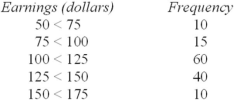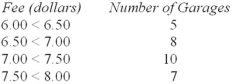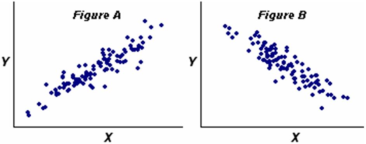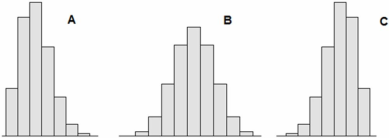/
/
/
115.The following frequency distribution shows the amount earned yesterday by
Not my Question
Flag Content

# Question : 115.The following frequency distribution shows the amount earned yesterday by

115.The following frequency distribution shows the amount earned yesterday by employees of a large Las Vegas casino. Estimate the mean daily earnings.A.\$112.50

B.\$125.01

C.\$105.47

D.\$117.13

116.The following table is the frequency distribution of parking fees for a day:The mean parking fee is:

A.\$7.07.

B.\$6.95.

C.\$7.00.

D.\$7.25.

117.Find the standard deviation of this sample: 4, 7, 9, 12, 15.

A.4.550

B.3.798

C.4.278

D.2.997

118.The 25th percentile for waiting time in a doctor's office is 10 minutes. The 75th percentile is 30 minutes. Which is incorrect regarding the fences?

A.The upper inner fence is 60 minutes.

B.The upper outer fence is 90 minutes.

C.A waiting time of 45 minutes would be an outlier.

D.The lower fences are not relevant in this problem.

119.Five homes were recently sold in Oxnard Acres. Four of the homes sold for \$400,000 while the fifth home sold for \$2.5 million. Which measure of central tendency best represents a typical home price in Oxnard Acres?

A.The mean or median.

B.The median or mode.

C.The mean or mode.

D.The midrange or mean.

120.In Tokyo, construction workers earn an average of ×420,000 (yen) per month with a standard deviation of ¥20,000, while in Hamburg, Germany, construction workers earn an average of €3,200 (euros) per month with a standard deviation of €57. Who is earning relatively more, a worker making ¥460,000 per month in Tokyo or one earning €3,300 per month in Hamburg?

A.The workers are the same in relative terms.

B.The Tokyo worker is relatively better off.

C.The Hamburg worker is relatively better off.

121.Which statement is false? Explain.

A.If μ = 52 and σ = 15, then X = 81 would be an outlier.

B.If the data are from a normal population, about 68 percent of the values will be within μ ± σ.

C.If μ = 640 and σ = 128, then the coefficient of variation is 20 percent.

122.Which is not a measure of variability?

B.Standard deviation

C.Midhinge

D.Interquartile range

123.If Q1 = 150 and Q3 = 250, the upper fences (inner and outer) are:

A.450 and 600.

B.350 and 450.

C.400 and 550.

124.Variables X and Y have the strongest correlation in which scatter plot?A.Figure A.

B.Figure B.

125.Which of the following statements is likely to apply to the incomes of 50 randomly chosen taxpayers in California?

A.The median income would probably be near the mean.

B.The midhinge would be a robust measure of center.

C.The sum of squared deviations about the mean would be negative.

D.Outliers in either tail would be equally likely.

126.A certain health maintenance organization (HMO) examined the number of office visits by each of its members in the last year. For this data set, we would anticipate that the geometric mean would be

A.a reasonable measure of center.

B.zero because some HMO members would not have an office visit.

C.too high because the distribution is likely to be skewed to the left.

D.negative because some data values would be below the mean.

127.Three randomly chosen Colorado students were asked how many times they went rock climbing last month. Their replies were 5, 6, 7. The coefficient of variation is:

A.16.7 percent.

B.13.6 percent.

C.20.0 percent.

D.35.7 percent.

128.The mean of a population is 50 and the median is 40. Which histogram is most likely for samples from this population?A.Sample A.

B.Sample B.

C.Sample C.

## Solution 5 (1 Ratings )

Solved
Statistics 8 Months Ago 4 Views